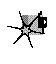In Listing 17.3, note the padded cellmap edges, and the alteration of the member functions to compensate for the padding. Also note that the width now has to be a multiple of eight, to facilitate the process of copying the edges to the opposite padding bytes. We have decreased the generality of our Game of Life implementation in exchange for better performance. That’s a very common trade-off, as common as trading memory for performance. As a rule, the more general a program is, the slower it is. A corollary is that often (not always, but often), the more heavily optimized a program is, the more complex and the more difficult to implement it is. You can often improve performance a good deal by implementing only the level of generality you need, but at the same time decreased generality makes it more difficult to change or port the program at some later date. A Game of Life implementation, such as Listing 17.1, that’s built on set_cell(), clear_cell(), and get_cell() is completely general; you can change the cell storage format simply by changing the constructor and those three functions. Listing 17.3 is harder to change because count_neighbors() would also have to be altered, and it’s more complex than any of the other functions.

So, in Listing 17.3, we’ve gotten under the hood and changed the cellmap format a little, and gotten impressive results. But now count_neighbors() is hard-wired for optimized counting, and it’s still taking up more than half the time. Maybe now it’s time to go to assembly?

Not hardly.

Heavy-Duty C++ Optimization

Before we get to assembly, we still have to perform C++ optimization, then see if we can find an alternative approach that better fits the application. It would actually have made much more sense if we had looked for a new approach as our first optimization step, but I decided it would be better to cover straightforward C++ optimizations at this point, and the mind-bending stuff a little later. Right now, let’s look at some C++ optimizations; Listing 17.4 is a C++-optimized version of Listing 17.3.

LISTING 17.4 L17-4.CPP

/* next_generation(), implemented using fast, all-in-one hard-wired
neighbor count/update/draw function. Otherwise, the same as
Listing 17.3. */

/* Calculates the next generation of current_map and stores it in
next_map. */
void cellmap::next_generation(cellmap& next_map)
{
unsigned int x, y, neighbor_count;
unsigned int width_in_bytesX2 = width_in_bytes << 1;
unsigned char *dest_cell_ptr = next_map.cells;

// Process all cells in the current cellmap
row_cell_ptr = cells;      // point to upper left neighbor of
// first cell in cell map
for (y=0; y<height; y++) { // repeat for each row of cells
// Cell pointer and cell bit mask for first cell in row
base_cell_ptr = row_cell_ptr; // to access upper left neighbor
base_mask = 0x01;             // of first cell in row
for (x=0; x<width; x++) {     // repeat for each cell in row
// First, count neighbors
// Point to upper left neighbor of current cell
cell_ptr = base_cell_ptr;  // pointer and bit mask for
// Count upper left neighbor
neighbor_count = (*cell_ptr & mask) ? 1 : 0;
// Count left neighbor
if ((*(cell_ptr + width_in_bytes) & mask)) neighbor_count++;
// Count lower left neighbor
if ((*(cell_ptr + width_in_bytesX2) & mask))
neighbor_count++;
// Point to upper neighbor
if ((mask >>= 1) == 0) {
cell_ptr++;
}
// Remember where to find the current cell
current_cell_ptr = cell_ptr + width_in_bytes;
// Count upper neighbor
// Count lower neighbor
if ((*(cell_ptr + width_in_bytesX2) & mask))
neighbor_count++;
// Point to upper right neighbor
if ((mask >>= 1) == 0) {
cell_ptr++;
}
// Count upper right neighbor
// Count right neighbor
if ((*(cell_ptr + width_in_bytes) & mask))  neighbor_count++;
// Count lower right neighbor
if ((*(cell_ptr + width_in_bytesX2) & mask))
neighbor_count++;
if ((neighbor_count != 2) && (neighbor_count != 3)) {
*(dest_cell_ptr + (current_cell_ptr - cells)) &=
draw_pixel(x, y, OFF_COLOR);
}
} else {
if (neighbor_count == 3) {
*(dest_cell_ptr + (current_cell_ptr - cells)) |=
draw_pixel(x, y, ON_COLOR);
}
}
// Advance to the next cell on row
if ((base_mask >>= 1) == 0) {
base_cell_ptr++;  // advance to the next cell byte
}
}
row_cell_ptr += width_in_bytes;  // point to start of next row
}
}

Listing 17.4 and Listing 17.3 are functionally the same; the only difference lies in how next_generation() is implemented. (Only next_generation() is shown in Listing 17.4; the program is otherwise identical to Listing 17.3.) Listing 17.4 applies the following optimizations to next_generation():

The neighbor-counting code is brought into next_generation, eliminating many function calls and from-scratch address/mask calculations; all multiplies are eliminated by using pointers and addition; and all cells are accessed directly via pointers and masks, eliminating all remaining function calls and from-scratch address/mask calculations.

The net effect of these optimizations is that Listing 17.4 is more than twice as fast as Listing 17.3; we’ve achieved the desired 18 generations per second, albeit only on a 486, and only at 96×96. (The #define that enables code limiting the speed to 18 Hz, which seemed ridiculous in Listing 17.1, is actually useful for keeping the generations from iterating too quickly when Listing 17.4 is running on a 486, especially with a small cellmap like 48×48.) We’ve sped things up by about eight times so far; we need to increase our speed another ten times to reach our goal of 200×200 at 18 generations per second on a 20 MHz 386.

It’s undoubtedly possible to improve the performance of Listing 17.4 further by fine-tuning the code, but no tremendous improvement is possible that way.Once you’ve reached the point of fine-tuning pointer usage and register variables and the like in C or C++, you’ve become compiler-dependent; you therefore might as well go to assembly and get the real McCoy.

Graphics Programming Black Book © 2001 Michael Abrash